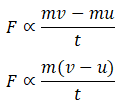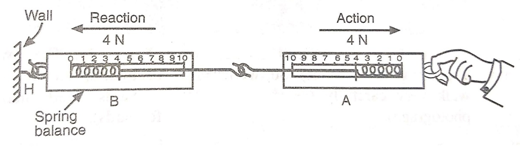# Newton’s laws of motion

Newton was a scientist who is acknowledged for his works in the field of science and mathematics. He has given three laws which govern the motion of bodies and forces acting upon them. These laws are known as Newton’s laws of motion.

These laws are used to know precisely about the force acting on the bodies and the relation between acting forces and the state of motion the body is in at any given time.

Let us discuss about these laws in detail one by one.

### Newton’s first law of motion –

We know that when an object does not change its position with respect to time it is said to be at rest and when an object covers equal distances in equal intervals of time it is said to be in uniform motion.

According to Newton’s first law of motion:“A body at rest will remain at rest and a body in uniform motion will continue its state of uniform motion in a straight line unless it is compelled by an external force to change its state of rest or of uniform motion”.

In the first law of motion external force is a force acting on the body from outside. The force acting from outside the body is only capable of bringing a change in its state of motion.

For example – a car moving on a straight road continues to move smoothly until brakes are applied. The brakes provide an external force that slows down the car and the state of motion of car changes from uniform motion to rest. Another example of this law can be a book placed on a table. The book is in rest and until we apply a force on the book to pick it up, it does not come in motion, hence only external force can change the state of motion of a body.

The tendency of a body to remain at rest or, if moving, to continue moving in a straight line is called inertia. Newton’s first law of motion is also referred to as Law of Inertia. Inertia is a property associated with the mass of a body. Greater the mass, greater will be the inertia of the body, greater will be the force required to bring a change in its motion.

Inertia can be experienced when suddenly brakes are applied in a car. Our upper body which is in motion continues to be in motion because no external force is applied on it but our lower body, which is in contact with the floor of car, experiences the force of brakes and comes to rest. Because of the incompatibility of the state of motion of the two halves of our body we experience a jerk.

### Newton’s second law of motion –

According to Newton’s second law of motion: “The rate of change of momentum of a body is directly proportional to the applied force, and takes place in the direction in which the force acts”.

The rate of change of momentum can be expressed by dividing change in momentum by the time taken for change.

Therefore, Newton’s second law can be expressed as

Let us consider a body of mass  having an initial velocity . Let us suppose a force   acts on the body for time and changes the velocity of body from u to v

Now, initial momentum of body = mu

Final momentum of the body = mv

Tie taken for change = t

Change in momentum = final momentum – initial momentum

= mv – mu

Substituting in above equation,But (v-u)/t represents change in velocity with time which is acceleration ‘’. So, we replace (v-u)/t by ‘a’ in above equation.

F ∝ m × a

F = k × m × a

In SI units, the value of  is equal to 1.

So, our final equation is –

F = m × a

Force = mass × acceleration

Hence, Newton’s second law gives a relation between force, mass and acceleration. It gives us a method to examine the effect of force on the acceleration of the body. The above equation can also be written as –

a = F/m

From this equation we can observe that acceleration of a body is directly proportional to the force applied and inversely proportional to the mass of body.

The SI unit of force is N (Newton) and from above equations we can see that

1 Newton = 1 kg × 1 m/s²

1 Newton = 1 kg m/s²

Example of second law – The players in a cricket match move their hands backwards after catching a ball because the velocity of the ball reduces to zero when it is caught. The players move their hands backwards to increase the time in which its velocity decreases to zero so that less force exerted by the ball on the hand of player. Another example is the seat belts provided in the car. During the time of accidents, the momentum of car reduces to zero in a very short time. The stretchable seat belts provide a longer time for the body to come to rest hence, decreasing the force on the human body.

### Newton’s third law of motion –

According to newton’s third law of motion: “Whenever one body exerts a force on another body, the second body exerts an equal and opposite force on the first body”.

The force exerted by the first body on the second body is called action and the force exerted by the second body on the first body is known as reaction.

Newton’s third law can also be stated as: “To every action there is an equal and opposite reaction”.

Action and reaction are two different forces but they act simultaneously on two different bodies.

This can be understood by the example of spring balances. If we join two spring balances with each other and pull on of them the reading in both of them will be same.The reading in both the balances is same because spring A applies a force of 4 N on spring B and according to Newton’s third law of motion spring B exerts an equal and opposite force on spring A.

### NCERT Solutions for Class 9 Science Chapter 8 – NCERT textbook

#### NCERT Solutions for Class 9 Science Chapter 8 – Page 118 (Newton’s laws of motion)

Question 1:

Which of the following has more inertia?

1. A rubber ball and a stone of the same size
2. A bicycle and a train
3. A five-rupee coin and a one-rupee coin

Answer:

1. A stone has more inertia than a rubber ball because it has more mass.
2. A train has more inertia than a bicycle because it has more mass than a bicycle
3. A fiverupee coin has more inertia than one-rupee coin because it has more mass.

Question 2:

In the following example, try to identify the number of times the velocity of the ball changes: “A football player kicks a football to another player of his team who kicks the football towards the goal. The goalkeeper of the opposite team collects the football and kicks it towards a player of his own team”. Also identify the agent supplying the force in each case.

Answer:

• First time, the velocity changes when player kicks the ball to his teammate.
• Second time, the velocity changes when the football is kicked towards the goal.
• Third time, the velocity changes when the goalkeeper stops the ball.
• Fourth time, the velocity changes when ball is kicked by the goalkeeper towards his teammate.

Question 3:

Explain why some of the leaves may get detached from a tree if we vigorously shake its branch.

Answer:

When we vigorously shake the branch of tree, force is applied on the branches and not on the leaves due to which the branch comes in motion but the leaves do not. Hence, they detach and fall down

Question 4:

Why do you fall in the forward direction when a moving bus brakes to a stop and fall backwards when it accelerates from rest?

Answer:

When a moving bus brakes to a stop our upper half of body continues to be in motion while the lower half comes in rest as it is in the contact with the floor of the bus. Hence, we fall forwards.

Similarly, when the bus starts moving from rest, the upper half of the body continues its state of rest and the lower half comes in motion. Hence, we fall backwards.

#### NCERT Solutions for Class 9 Science Chapter 8 – Page 126 (Newton’s laws of motion)

Question 1:

If action is always equal to reaction, explain how a horse can pull a cart.

Answer:

To pull a cart horse applies a force on the cart and in return the cart also applies an equal force on the horse. These forces act simultaneously but act on different bodies. The force that is applied on the horse determines the movement of horse, not the force it exerts. Hence, if the horse is able to apply enough force to overcome friction, the cart will move.

Question 2:

Explain why it is difficult for a fireman to hold a hose which ejects large amounts of water at a high velocity?

Answer:

When a hose pipe ejects large amounts of water, the flow of water exerts a force on the pipe in forwards direction.  According to Newton’s third law, the pipe exerts an equal amount of force in the backwards direction due to which the pipe tends to go backwards and slips from the fireman’s hands.

#### NCERT Solutions for Class 9 Science Chapter 8 – Page 128 (Newton’s laws of motion)

Question 2:

When a carpet is beaten with a stick, dust comes out of it. Explain.

Answer:

When a carpet is beaten with a stick, the carpet comes in motion but the dust particles remain at rest. Therefore, they detach from the carpet and come out.

Question 3:

Why is it advised to tie any luggage kept on the roof of a bus with a rope?

Answer:

It is advised to tie the luggage kept on the roof of a bus with rope because when the bus suddenly starts moving, the luggage will continue to be at rest and can fall down. Similarly, when the bus stops suddenly, the luggage does not come to rest and may fall down from the bus.

Question 4:

A batsman hits a cricket ball which then rolls on a level ground. After covering a short distance, the ball comes to rest. The ball slows to a stop because

• the batsman did not hit the ball hard enough.
• velocity is proportional to the force exerted on the ball.
• there is a force on the ball opposing the motion.
• there is no unbalanced force on the ball, so the ball would want to come to rest.

Answer:

(c) there is a force on the ball opposing the motion.

Newton was a scientist who did immense work in the field of science and mathematics. Newton’s laws of motion are useful in physics to analyse various natural phenomena. These laws and their associated concepts together explain many observations such as the way humans walk, rocket propulsion, the recoiling of gun, use of seat belts etc. The first law of motion explains the concept of inertia of bodies. Second law of motion gives an equation to establish the relation between force, mass and acceleration. The third law explains action-reaction pair that we observe around us very commonly.

These laws are capable to explain any natural phenomena sufficiently and are the basis of more advanced concepts of physics.

Click here for NCERT Solutions for Class 9